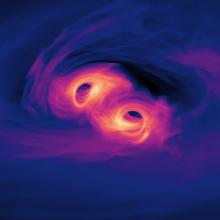Quasi-Periodicity of Supermassive Binary Black Hole Accretion
By Dennis B. Bowen, Vassilios Mewes, Scott C. Noble, Mark Avara, Manuela Campanelli, and Julian H. Krolik
Published in The Astrophysical Journal 879 No2 (Thursday, May 2, 2019)

## Abstract

In this paper we continue the first ever study of magnetized mini-disks
coupled to circumbinary accretion in a supermassive binary black hole (SMBBH)
approaching merger reported in Bowen et al. 2018. We extend this simulation
from 3 to 12 binary orbital periods. We find that relativistic SMBBH accretion
acts as a resonant cavity, where quasi-periodic oscillations tied to the the
frequency at which the black hole's orbital phase matches a non-linear $m=1$
density feature, or lump'', in the circumbinary accretion disk permeate the
system. The rate of mass accretion onto each of the mini-disks around the black
holes is modulated at the beat frequency between the binary frequency and the
lump's mean orbital frequency, i.e., $\Omega_{\rm beat} = \Omega_{\rm bin} - \bar{\Omega}_{\rm lump}$, while the total mass accretion rate of this
equal-mass binary is modulated at two different frequencies, $\gtrsim \bar{\Omega}_{\rm lump}$ and $\approx 2 \Omega_{\rm beat}$. The instantaneous
rotation rate of the lump itself is also modulated at two frequencies close to
the modulation frequencies of the total accretion rate, $\bar{\Omega}_{\rm lump}$ and $2 \Omega_{\rm beat}$. Because of the compact nature of the
mini-disks in SMBBHs approaching merger, the inflow times within the mini-disks
are comparable to the period on which their mass-supply varies, so that their
masses---and the accretion rates they supply to their black holes---are
strongly modulated at the same frequency. In essence, the azimuthal symmetry of
the circumbinary disk is broken by the dynamics of orbits near a binary, and
this $m=1$ asymmetry then drives quasi-periodic variation throughout the
system, including both accretion and disk-feeding. In SMBBHs approaching
merger, such time variability could introduce distinctive, increasingly rapid,
fluctuations in their electromagnetic emission.

## CCRG Authors

Campanelli, Manuela